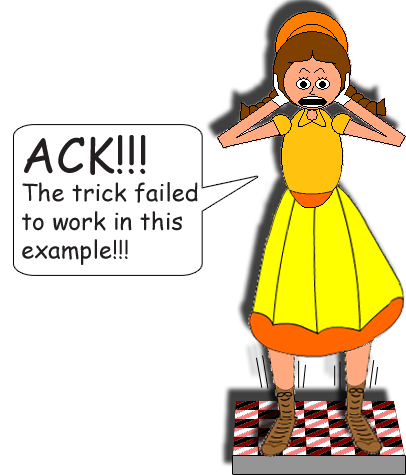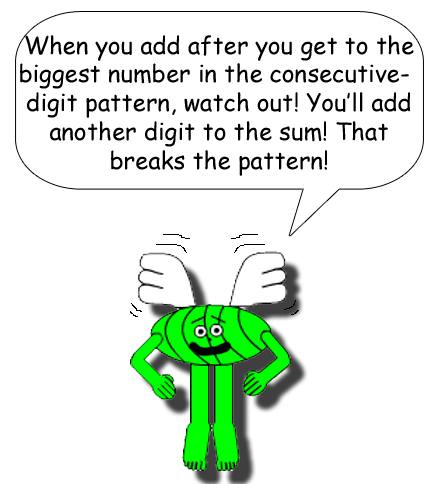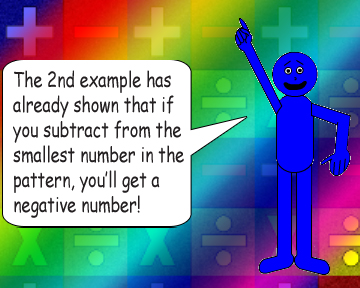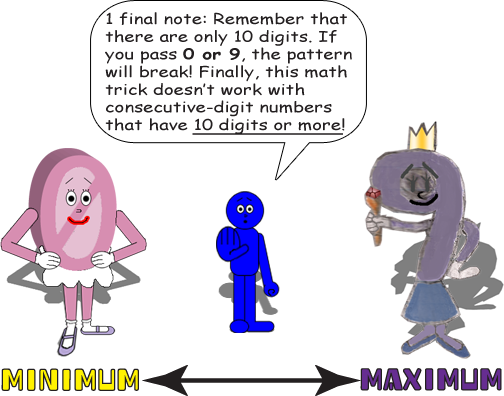# Consecutive Digit Slide

## Examples:

01 + 11 = 12

12 + 11 = 23

23 + 11 = 34

34 + 11 = 45

45 + 11 = 56

56 + 11 = 67

67 + 11 = 78

78 + 11 = 89

[The pattern stops here because if you add 11 to 89, you'll get 100]

Did you notice the padding zero I put for the number 1 on the top? That was so it would have 2 digits!

## Let's put the greater digit on the left this time!

98 - 11 = 87

87 - 11 = 76

76 - 11 = 65

65 - 11 = 54

54 - 11 = 43

43 - 11 = 32

32 - 11 = 21

21 - 11 = 10

[The pattern stops here because if you subtract 11 from 10, you'll get -1]

There must be a 1 for each digit column when you add or subtract consecutive-digit numbers for this trick to work...

012 + 111 = 123

123 + 111 = 234

234 + 111 = 345

345 + 111 = 456

987 - 111 = 876

876 - 111 = 765

765 - 111 = 654

654 - 111 = 543

...Otherwise, something like this will happen!

345 + 101 = 446## Here's the backwards version of the 1st example!

12 - 11 = 01

23 - 11 = 12

34 - 11 = 23

45 - 11 = 34

56 - 11 = 45

67 - 11 = 56

78 - 11 = 67

89 - 11 = 78

[If you subtract 11 from 1, you'll get -10]

Subtracting instead of adding gives you the previous, smaller consecutive-digit number in the pattern! (If the greater/greatest digit is on the right!)

98 + 11 = 109

87 + 11 = 98

76 + 11 = 87

65 + 11 = 76

54 + 11 = 65

43 + 11 = 54

32 + 11 = 43

21 + 11 = 32

10 + 11 = 21

[Adding 11 to 98 gave us a 3-digit number!]

If you add instead of subtract with the greater/greatest digit on the left, you get the next, bigger consecutive-digit number in the pattern!## Final Example:

12345 + 11111 = 23456

12345 - 11111 = 01234

54321 + 11111 = 65432

54321 - 11111 = 43210

See how the digits "slide" thru the sums? Cool, isn't it?